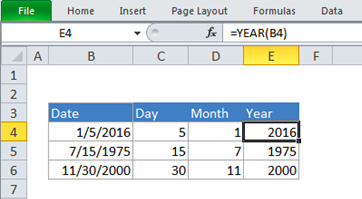Get instant live expert help with Excel or Google Sheets“My Excelchat expert helped me in less than 20 minutes, saving me what would have been 5 hours of work!”

#### Post your problem and you’ll get expert help in seconds.

Your message must be at least 40 characters
Our professional experts are available now. Your privacy is guaranteed.

# How to get a year from date in Excel – Excelchat

Are you wondering how to extract year from date in an Excel worksheet? Well, this should not be a difficult task. As we work on Excel sheets, time comes when we have to get the year from dates in order to arrange occurrences based on the year they occurred. This can easily be done using the Excel YEAR function as we shall shortly see in this post.Figure 1: How to get year from date in Excel

## How to use the date to year formula in Excel

As already mentioned, we can utilize the Excel formula date to year in order to extract the year from a given date in Excel. The formula is as shown below;

## General year to date formula

`=YEAR (A1)`

Where A1 represents the cell with the date from which we want to extract the year.

For the above formula to work correctly and help you convert date to year in Excel, the date must first be in a format that Excel recognizes as a valid date. Note that you can format values in Excel sheets into Excel recognizable date formats.

As you can see from the above formula and figure 1, the Excel date year formula only takes one argument, the date.

Note that in the example in figure 1 above, cell B4 has the date 1/5/2016. It is therefore correct to use the cell B4 as our reference cell. When we apply the formula, we are able to extract the year from the date. The year is simply 2016. This is done as below;

`=YEAR (B4)`

It is important to note that we can use the YEAR function when working on dates that have just been entered as text. For example, in the above example in figure 1, we shall still get the same result, 2016, if we wrote the formula as below;

`=YEAR (“1/5/2016”)`

But it is equally important to note that using text for dates can cause unpredictable results. This is due to the differences in regional date settings. For this reason, it is always advisable to use a cell reference with a valid date.

For those who might want to supply a whole date but only display the year, then it is good to understand how to apply a custom number format for that. The format is simply as “yyyy” or “yy”.